Function Repository Resource:

# SpringPendulumFormula

Compute the final angle and spring length of a spring pendulum based on initial conditions

Contributed by: Jason Martinez
 ResourceFunction["SpringPendulumFormula"][params,cond] computes the angle and spring length based on system parameters params and initial conditions cond. ResourceFunction["SpringPendulumFormula"][property] returns the specified property of the spring pendulum formula.

## Details and Options

To solve for the final angle and length of a spring pendulum, the following system parameters must be defined in params:
 k "k" spring constant m "m" mass l0 "l0" spring equalibrium length
Gravitational acceleration "g" can also be specified. If unspecified, it is assumed to be equal to "StandardAccelerationOfGravity".
Additionally, the following initial conditions must be defined in cond:
 li "li" initial spring length t "t" final time θi "thetai" initial angle from vertical
Parameters can use the keys provided in the second columns or the full QuantityVariable format shown by ResourceFunction["SpringPendulumFormula"]["QuantityVariables"].
In addition to the final spring length and angle, the solutions for the angle and length evolutions ("AngleSolution" and "LengthSolution") from the t=0 to the final time t are provided.
Properties include:
 "Formula" equations for spring pendulum "QuantityVariableDimensions" list of base dimensions for all variables "QuantityVariableNames" English names for all variables "QuantityVariablePhysicalQuantities" physical quantities for all variables "QuantityVariables" list of all variables "QuantityVariableTable" details on all variables

## Examples

### Basic Examples (2)

Solve for the final spring length and final angle from the vertical for a spring pendulum:

 In:=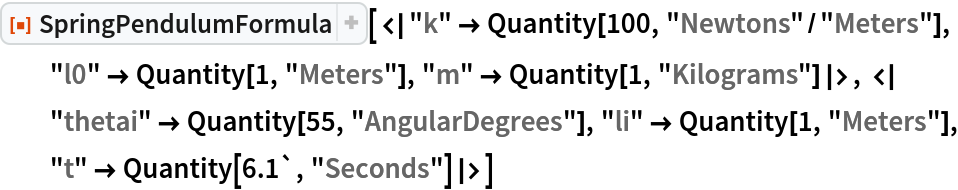Out=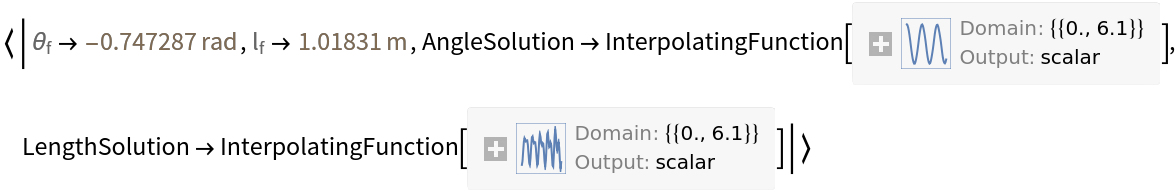Specify gravitational acceleration:

 In:=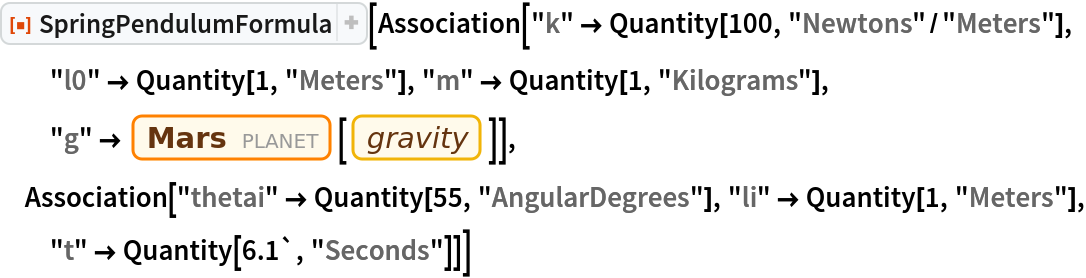Out=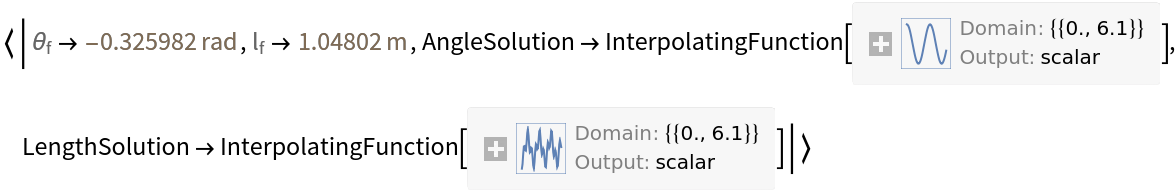### Scope (3)

Examine the equations of motion for a spring pendulum:

 In:=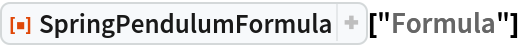Out=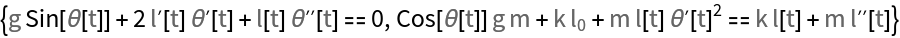Find the quantity variables used by the SpringPendulumFormula:

 In:=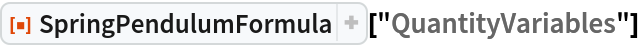Out=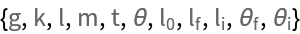Obtain their formal names:

 In:=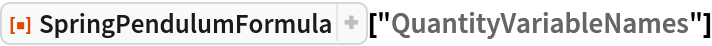Out=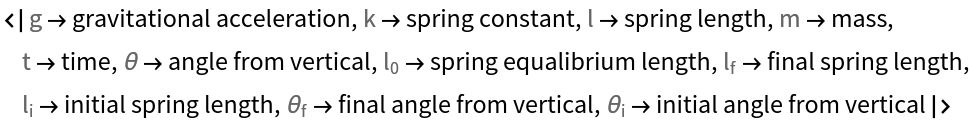Derive the physical quantities and unit dimensions of the variables:

 In:=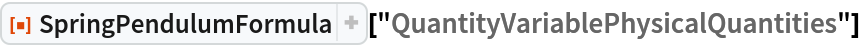Out=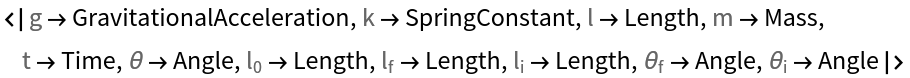In:=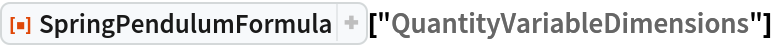Out=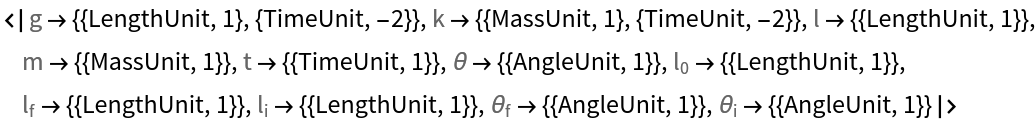Examine a table combining all the information about the quantity variables used or derived by SpringPendulumFormula:

 In:=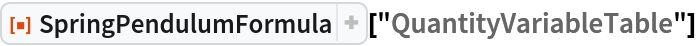Out=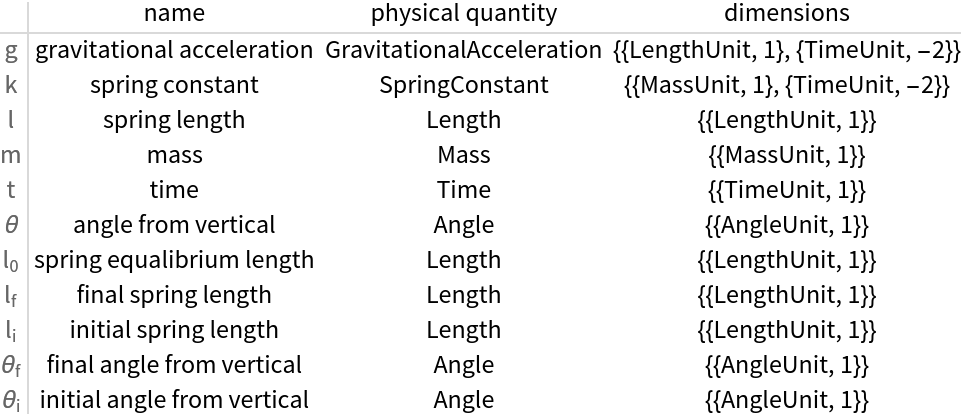### Applications (1)

Plot angle and length evolution over time:

 In:=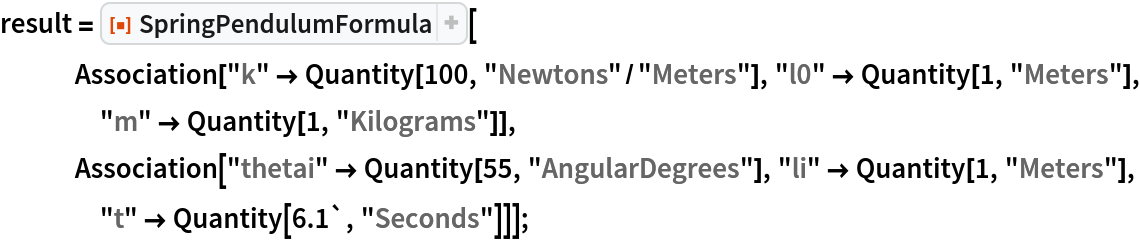In:=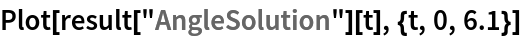Out=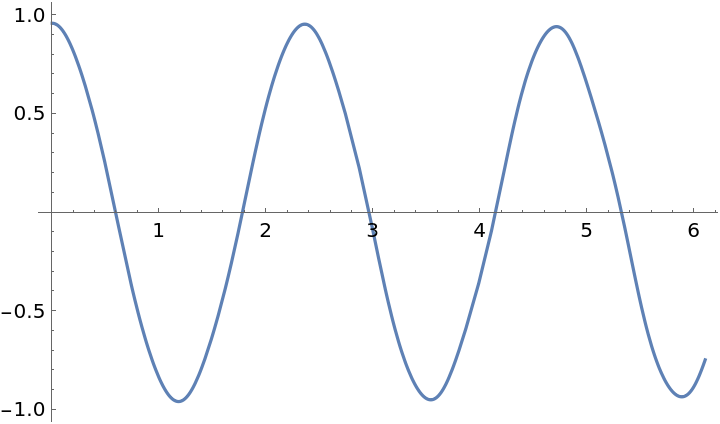In:=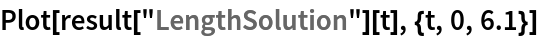Out=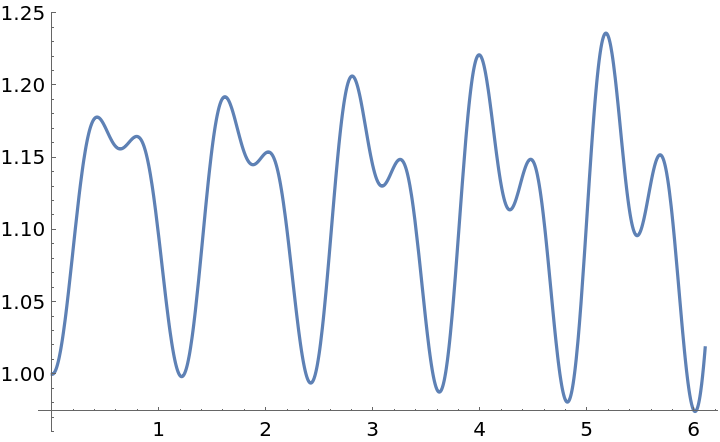## Version History

• 1.0.0 – 04 November 2019